# Important Questions CBSE Class 9 Maths Chapter 5-Introduction to Euclid's Geometry)

CBSE Class 9 Maths important questions with solutions for chapter 5-Introduction to Euclid’s Geometry are given here. These questions are based on CBSE syllabus (2022-2023) and NCERT book. All the questions are prepared as per the latest exam pattern. Students who want to prepare and revise this chapter for the final exam could practice these problems. These materials are prepared by our subject experts and are helpful for students to score good marks. To get chapter-wise important questions for Class 9 Maths with solutions reach us at BYJU’S. Here we are also providing a few extra questions based on exam papers, to make students prepare well.

Also Check:

## Important Questions & Solutions for Class 9 Maths Chapter 5 (Introduction to Euclid’s Geometry)

Q.1: What are the five postulates of Euclid’s Geometry?

1. A straight line may be drawn from one point to any other point.
2. A terminated line can be produced indefinitely.
3. A circle can be drawn with any centre and any radius.
4. All right angles are equal to one another.
5. If a straight line falling on two straight lines makes the interior angles on the same side of it taken together less than two right angles, then the two straight lines if produced indefinitely, meet on that side on which the sum of angles is less than two right angles.

Q.2: If a point C lies between two points A and B such that AC = BC, then prove that AC =1/2 AB. Explain by drawing the figure.

Solution: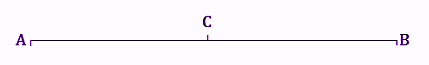Given, AC = BC

Now, add AC on both sides.

L.H.S + AC = R.H.S + AC

AC + AC = BC + AC

2AC = BC + AC

Since, we know,

BC +AC = AB (as it coincides with line segment AB, from figure)

∴ 2 AC = AB (If equals are added to equals, the wholes are equal.)

⇒ AC = 1/2 AB.

Q.3: If in Q.2, point C is called a mid-point of line segment AB. Prove that every line segment has one and only one mid-point.

Solution: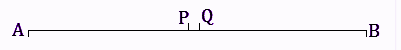Let, AB be the line segment

Assume that points P and Q are the two different midpoints of AB.

Therefore,

AP=PB ………(1)

and AQ = QB …..(2)

Also,

PB + AP = AB (as it coincides with line segment AB)

Similarly, QB + AQ = AB.

Now,

Adding AP to the L.H.S and R.H.S of the equation (1)

We get, AP + AP = PB + AP (If equals are added to equals, the wholes are equal.)

⇒ 2 AP = AB — (3)

Similarly,

2 AQ = AB — (4)

From (3) and (4),

2 AP = 2 AQ

⇒ AP = AQ

Thus, we conclude that P and Q are the same points.

This contradicts our assumption that P and Q are two different midpoints of AB.

Thus, it is proved that every line segment has one and only one mid-point.

Hence Proved.

Q.4: In the given figure, if AC = BD, then prove that AB = CD.

Solution: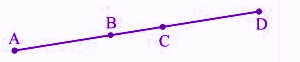It is given, AC = BD

From the given figure, we get,

AC = AB + BC

BD = BC + CD

⇒ AB + BC = BC + CD [Given: AC=BD]

We know that, according to Euclid’s axiom, when equals are subtracted from equals, remainders are also equal.

Subtracting BC from the L.H.S and R.H.S of the equation AB + BC = BC + CD, we get,

AB + BC – BC = BC + CD – BC

AB = CD

Hence proved.

Q.5: Does Euclid’s fifth postulate imply the existence of parallel lines? Explain.

Solution:

Yes, Euclid’s fifth postulate does imply the existence of the parallel lines.

If the sum of the interior angles is equal to the sum of the right angles, then the two lines will not meet each other at any given point, hence making them parallel to each other.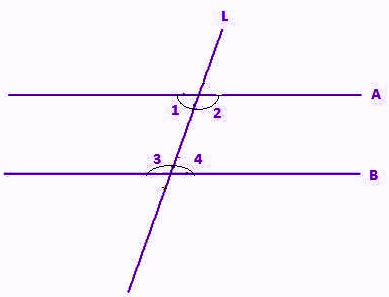So,

∠1+∠2=180°

Or ∠3+∠4=180°

Q.6: It is known that x + y = 10 and that x = z. Show that z + y = 10.

Solution:

According to the question,

We have,

x+y=10 …(i)

And, x=z …(ii)

Applying Euclid’s axiom,

“if equals are added to equals, the wholes are equal”

We get,

From Eq. (i) and (ii)

x+y=z+y ….(iii)

From Eqs. (i) and (iii)

z+y=10

## Extra Questions for Class 9 Maths Chapter 5 – For Practice

1. Write whether the following statements are True or False? Justify your answer:
• Euclidean geometry is valid only for curved surfaces.
• The boundaries of the solids are curves.
• The edges of a surface are curves.
• The things which are double of the same thing are equal to one another.

2. Two salesmen make equal sales during the month of August. In September, each salesman doubles his sale of the month of August. Compare their sales in September.

3. Look at the figure below. Show that length AH > sum of lengths of AB + BC + CD.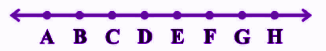4. Prove that an equilateral triangle can be constructed on any given line segment.

5. Ravi and Ketan have the same weight. If they each gain weight by 2 kg, calculate the comparison between their new weights.

6. Given that a + b = 10 then a + b + c = 10 + c. Then which of the Euclid’s axiom illustrates this statement?

(a) 1st Axiom

(b) 2nd Axiom

(c) 3rd Axiom

(d) 4th Axiom